## How to use SOH CAH TOA: Geometry

Greeting math friends and welcome to Mathsux! In this post, we are going to start with the very basics of trigonometry by going over how to find a missing angle and/or side length of right triangles while using the famous trigonometric function sine, cosine, or tangent, (aka how to use SOH CAH TOA).  Woo hoo! These are the basics of right triangle trigonometry, and provide the base for mastering so many more interesting things to come in trigonometry! So, let’s get to it!

SOH CAH TOA is an acronym that stands for the following trig functions and parts of a right triangle. We’ll explain more in this post!

SOH CAH TOA

Also, if you have any questions about anything here, don’t hesitate to comment below or watch the video below. Also, don’t forget to subscribe to MathSux for FREE math videos, lessons, and practice questions every week. Happy calculating! 🙂

## What does SOH CAH TOA stand for?

A Trigonometric Ratio, more commonly known as Sine, Cosine, and Tangent, are trig ratios that naturally exist within a right triangle.  This means that the sides and angles of a right triangle are in proportion within itself.  It also means that if we are missing a side or an angle of a right triangle, based on what we’re given, we can figure out what the value of the sides or angles are, based on these ratios!

Let’s take a look at what Sine, Cosine, and Tangent are all about!

Ready for your first right angled triangle example? Check it out below!

## SOH CAH TOA Example #1:

Now let’s see how we can apply trig ratios when there is a missing side or angle in a right triangle!

Step 1: First, let’s identify the different sides of our right triangle depending on which angle we are focusing on, which in this case is a 60º angle. Based on the locations of our angle, we can label each side as the hypotenuse, adjacent, or the opposite.

Notice below, that the opposite side labeled x, is labeled the “opposite” side because it is opposite to our given angle, 60º. The remaining side is considered the adjacent side of our triangle because it is directly next to our given angle, 60º.

Step 2: Now, let’s write out SOH CAH TOA. Notice the only trig function that uses both the hypotenuse and the opposite is sine! Knowing to use the sine function, let’s fill in our formula using the hypotenuse = 5 and opposite = x in order to find the value for missing side length x.

In order to use the sin function correctly, we’re going to need to plug in our given angle, which is 60º, and then set up our proportion. sin(60º)=x/5. By using the sine function, our calculator, and a little bit of algebra we’ll be able to solve for the unknown side.

Ready for another example?! Check out another SOH CAH TOA problem using right triangles below!

## Right Triangle Trig Example #2:

Step 1: First, let’s identify the different parts of the right triangle we are given (the hypotenuse, adjacent, and the opposite). Notice in this example, we are given the adjacent and hypotenuse and need to find the value of the unknown angle, θ.

Step 2: Next, let’s write out our acronym, SOH CAH TOA, to see which trig function can help us with our question! Notice the only trig function that uses both adjacent and hypotenuse is cosine. This is what we will use to solve for the unknown angle, θ.

We use cosine, by setting up our proportion, cos(θ)=adjacent/ hypotenuse, knowing we can then plug in 12 for our adjacent value, and 13 for our hypotenuse value.

Think you’re ready to test out SOH CAH TOA on your own? Try the following Practice Questions on your own!

## Practice Questions:

Given the following right triangles, find the missing lengths and side angles rounding to the nearest whole number.

## Solutions:

Still got questions?  No problem! Check out the video the same examples outlined above and happy calculating! 🙂

## Related Trigonometry Posts:

The Unit Circle

Basic Right Triangle Trigonometric Ratios (SOH CAH TOA)

4545 90 Special Triangles

30 60 90 Special Triangles

Graphing Trig Functions

Transforming Trig Functions

Factoring Trig Functions

Law of Cosines

Trig Identities

Law of Sines

## How to Solve Log Equations: Algebra 2/Trig.

Welcome to Mathsux! Today, we’re going to go over how to solve log equations, yay! But before we get into finding x, though, we need to go over what log equations are and why we use them in the first place…..just in case you were curious!

Also, if you have any questions about anything here, don’t hesitate to comment below or shoot me an email.  Happy calculating! 🙂

## What are Log Equations?

Logarithms are the inverses of exponential functions.  This means that when graphed, they are symmetrical along the line y=x.  Check it out below!

When on the same set of axis, notice how the functions are symmetrical over the line y=x:

We use logarithms to find the unknown values of exponents, such as the x value in the equation,.  This is a simple example, where we know the value of x is equal to 2,(). But what if it were to get more complicated?  That’s where logs come in!

## How to Solve Log Equations?

Logarithms follow a swooping pattern that allows us to write it in exponential form, let’s take a look at some Examples below:

But wait there’s more! Logs have a set of Rules that makes solving log equations a breeze!

We can use these rules to help us algebraically solve logarithmic equations, let’s look at an example that applies the Product Rule.

## Example:

Try the following practice questions on your own!

Practice Questions:

Solutions:

Still got questions?  No problem! Check out the video that goes over the same example outlined above.  And for more info. on logarithms check out this post that goes over a NYS Regent’s question here.  Subscribe below to get the latest FREE math videos, lessons, and practice questions from MathSux. Thanks for stopping by and happy calculating! 🙂

****Check out this Bonus Video on How to Change Log Bases****

## Median of a Trapezoid Theorem: Geometry

Hi everyone and welcome to Math Sux! In this post, we are going to look at how to use and applythe median of a trapezoid theorem. Thankfully, it is not a scary formula, and one we can easily master with a dose of algebra. The only hard part remaining, is remembering this thing! Take a look below to see a step by step tutorial on how to use the median of a trapezoid theorem and check out the practice questions at the end of this post to truly master the topic. Happy calculating! 🙂

*If you haven’t done so, check out the video that goes over this exact problem, also please don’t forget to subscribe!

Step 1:  Let’s apply the Median of a Trapezoid Theorem to this question!  A little rusty?  No problem, check out the Theorem below.

Median of a Trapezoid Theorem: The median of a trapezoid is equal to the sum of both bases.Step 2: Now that we found the value of x , we can plug it back into the equation for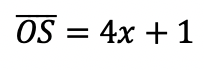median,  to find the value of median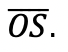Want more practice?  Your wish is my command! Check out the practice problems below:

Practice Questions:

1.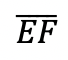is the median of trapezoid ABCDEF, find the value of the median, given the following:2.is the median of trapezoid ACTIVE, find the value of the median, given the following:3.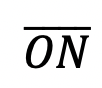is the median of  trapezoid DRAGON, find the value of the median, given the following:

4.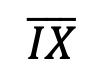is the median of trapezoid MATRIX, find the value of the median, given the following:

Solutions:

Need more of an explanation?  Check out the detailedand practice problems. Happy calculating! 🙂

## Dividing Polynomials: Algebra 2/Trig.

Greeting math peeps! In this post we are going to go over dividing polynomials! At some point, you may need to know how to answer these types of questions. The cool thing about dividing polynomials is that it’s the same long division you did way back in grade school (except now with a lot of x). Ok, let’s get to it and check out the question below:

Also, if you haven’t done so, check out the video related that corresponds to this problem on Youtube below! 🙂

Explanation:

How do I answer this question?

The question wants us to divide polynomials by using long division.

How do we do this?

Step 1: First we set up a good ole’ division problem with the divisor, dividend, and quotient to solve.

Step 2: Now we use long division like we used to back in the day! If you have any confusion about this please check out the video in this post.

## What if there’s a Remainder?

What happens when there is a remainder though!?!? When dividing polynomials with a remainder in the quotient, the answer is found and checked in a very similar way! Check it out in the example below:

Notice we represented the remainder by adding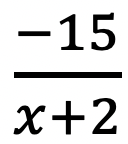to our quotient! We just put the remainder over the divisor to represent this extra bit of solution.

Want more practice? Try solving these next few examples on your own.

## Solutions:

If you’re looking for more on dividing polynomials, check out this post on synthetic division and finding zeros here!

Still got questions? No problem! Don’t hesitate to comment with any questions or check out the video above. Happy calculating! 🙂

## Perpendicular Lines through a Given Point: Geometry

Ahoy math peeps! I’m writing this during the time of the coronavirus, and although, the NYS Regents tests may be canceled, online zooming is still on! From the good ole’ days of test-taking and sitting in a giant room together, I bring to you a Regent’s classic, a question about how to find perpendicular lines through a given point. We will go over the following Regents question, starting with a review of what perpendicular lines are. Stay curious and happy calculating! 🙂

Perpendicular Lines: When two lines going in opposite directions come together to form a perfect 90º angle! Sounds magical, am I right? Check it out for yourself below:

A super exciting feature of these so-called perpendicular lines is that their slopes are negative reciprocals of each other. Wait, what?

How do we do this? Now it is time to go back and answer our question!

First, our equation 2y+3x=1 looks kind of cray, so let’s get it back to normal in y=mx+b form:

Need more of an explanation? Check out the video that goes over these types of questions up on Youtube (video at top of post) and let me know if you have still any questions.

Happy Calculating! 🙂

Looking for more on Perpendicular and parallel lines? Check out the difference between the two in this post here!

## COVID-19: What does #FlattenTheCurve even mean?

COVID-19: What does #FlattenTheCurve even mean? If you are a human on Earth, then I’m sure you’ve heard about the coronavirus and are currently social distancing. Here in NYC, I’m quarantining like everyone else and listening to all the beautiful math language that has suddenly become mainstream (so, exciting)!  #FlattenTheCurve has become NY’s new catchphrase and for anyone confused about what that means, you’ve come to the right place!

The coronavirus spreads at an Exponential Rate, which means it spreads in a way that increases faster and faster every day.

What does this mean?

For Example, one person with the virus can easily spread the virus to 5 other people, those 5 people can then spread the virus to another 5 people each for a total of an extra 25 people, these 25 people can then spread it to another 5 people each for an extra 125 infected people! And the pattern continues……. See below to get a clearer picture:

.   *Note: These numbers are not based on actual coronavirus data

The Example we just went over is equal to the exponential equation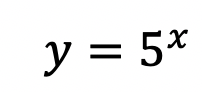, but it is only that, an Example! The exact pattern and exponential equation of the future progress of the virus is unknown! We mathematicians, can only measure what has already occurred and prepare/model for the future.  To make the virus spread less rapidly, it is our duty to stay home to slow the rate of this exponentially spreading virus as much as possible.

We want to #FlattenTheCurve a.k.a flatten the increasing exponential curve of new coronavirus cases that appear every day! Hopefully, this post brings some clarity to what’s going on in the world right now.  Even with mathematics, the true outcome of the virus may be unknown, but understanding why we are all at home in the first place and the positive impact it has is also important (and kind of cool).

Stay safe math friends and happy calculating! 🙂

Want to make math suck just a little bit less? Subscribe and follow us for FREE fun colorful math videos and lessons every week! 🙂

## Math Resources (in the time of COVID)

Calling all students, teachers, and parents!  As everyone is stuck at home during a global pandemic, now is a great time we are all forced to try and understand math (and our sanity level) a little bit more.  Well, I may not be able to help you with the keeping sanity stuff, but as far as math goes, hopefully, the below math resources offer some much needed mathematic support.

All jokes aside I hope everyone is staying safe and successfully social distancing.  Stay well, math friends! 🙂

Kahn Academy: The same Kahn Academy we know and love still has amazing videos and tutorials as usual, but now they also have a live “homeroom” chat on Facebook LIVE every day at 12:00pm. The chats occur daily with Kahn Academy founder Sal and at times feature famous guests such as Bill Gates. Click the link below for more:

Study.com: In a time when companies are being more generous, Study.com is here for us as they offer up to 1000 licenses for school districts and free lessons for teachers, students, and parents.  Check out all the education freebies here:

Study.com

Math PlanetIf you’re looking for free math resources in Pre-Algebra, Algebra, Algebra 2, and Geometry then you will find the answers you need at Math Planet.  All free all the time, find their website here:

MathSux: Clearly, I had to mention MathSux, the very site you are on right now! Check out free math videos, lesson, practice questions, and more for understanding math any way that works for you!

MathSux

What is your favorite educational site?  Let me know in the comments, and stay well! 🙂

## Binomial Cubic Expansion: Algebra 2/Trig.

Hey math friends! In this post, we are going to go over Binomial Cubic Expansion by going step by step! We’ll start by reviewing an old Regents question. Then, to truly master the topic, try the practice problems at the end of this post on your own! And, if you still have questions, don’t hesitate to watch the video or comment below. Thanks for stopping by and happy calculating! 🙂

Also, if you’re looking for more on Binomial Cubic Expansion, check out this post here!

## What are Cubed Binomials?

Binomials are two-termed expressions, and now we are cubing them with a triple exponent! See how to tackle these types of problems with the example below:

How do I answer this question?

We need to do an algebraic proof to see if (a+b)3=a3+b3.

How do we do this?

We set each expression equal to one another, and try to get one side to look like the other by using FOIL and distributing. In this case, we will be expanding (a+b)3 to equal (a+b)(a+b)(a+b).

Extra Tip! Notice that we used something called FOIL to combine (a+b)(a+b).  But what does that even mean? FOIL is an acronym for multiplying the two terms together.  It’s a way to remember to distribute each term to one another.  Take a look below:

Add and combine all like terms together and we get!

_______________________________________________________________________

Keep in touch with MathSux and get FREE math videos, lessons, and practice questions straight to your inbox! Thanks for stopping by and happy calculating! 🙂

## Volume of a Cone: Geometry

The Voluminous “Vessel” at Hudson Yards

Calling all NYC dwellers! Have you seen the new structure at Hudson Yards?  A staircase to nowhere, this bee-hive like structure is for the true adventurists at heart; Clearly, I had to check it out!

Where does math come in here you say?  Well, during my exploration, I had to wonder (as am sure most people do) what is the volume of this almost cone-like structure? It seemed like the best way to estimate the volume here, was to use the formula for the volume of a cone!

## Volume of a Cone:

I estimated the volume by using the formula of a three-dimensional cone. (Not an exact measurement of the Vessel, but close enough!) .

We can find the radius and height based on the given information above.  Everything we need for our formula is right here!

Now that we have our information, let’s fill in our formula and calculate!

Extra Tip! Notice that we labeled the solution with feet cubed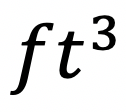, which is the short-handed way to write “feet cubed.”  Why feet cubed instead of feet squared? Or just plain old feet? When we use our formula we are multiplying three numbers all measured in feet:

All three values are measured in feet! –> Feet cubed ()

____________________________________________________________________________________

Did you get the same answer? Did you use a different method or have any questions?  Let me know in the comments and happy calculating! 🙂

Looking to apply more math to the real world? Check out this post on the Golden Ratio here!

## Rate of Change: Algebra

The rate of change, the rate of motion, the rate of a heartbeat. A chart on a piece of paper as boring as it may seem, can produce some pretty great numbers that directly relate to us human folk.

The question below may make you groan at first glance, but what happens if we use our imagination to picture the real-life bird it’s describing? Any better? Yes? No? Well, either way we must solve, so let’s get to it

## How do I answer this question?

It wants us to find the Rate of Change, specifically between 3 and 9 seconds. Let’s hi-light those two values on our given table:

## What is the Rate of Change?

To get the Rate of Change between these two values, we need to go back to the good ole’ Slope Formula and realize that the list of values is really a list of x and y coordinates.

Now let’s plug in the coordinate values (3, 6.26) and (9,3.41) into our slope formula:

Extra Tip! Notice that we added the labels feet/second to our answer.  Why does this make sense?? The question tells us that P(t) represents feet and that t is equal to seconds.  Another way to look at this question when applying it to the slope formula is to realize that we are finding the change of feet divided by the change of seconds.                                                          ____________________________________________________________________________________

Still got questions?  Let me know in the comments and as always happy calculating!:)

Looking for the next step? Learn how to graph equation of a line, y=mx+b here!